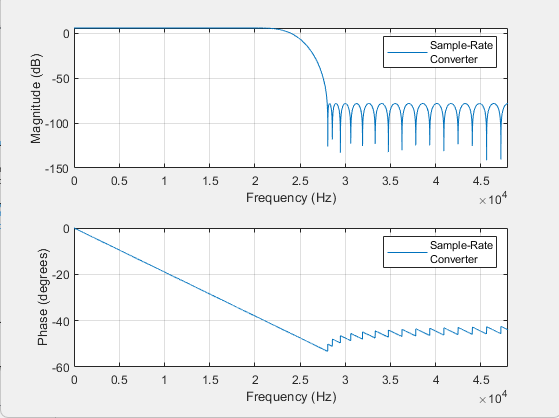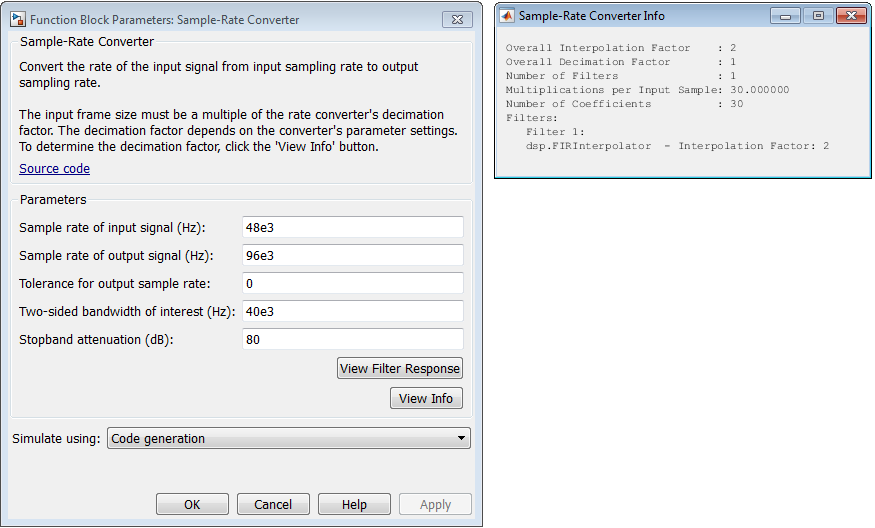# Sample-Rate Converter

Multistage sample-rate conversion

## Library

Signal Operations

`dspsigops`

•## Description

The Sample-Rate Converter block implements a multistage FIR sample-rate converter. This multistage FIR converter converts the rate of each channel of the input signal from the input sample rate to the output sample rate. Multistage implementations minimize the amount of computation required by the sample-rate conversions by first reducing the sample rate of the input signal. Next, the block determines the optimal number of decimators and interpolators required based on the parameters specified in the block dialog box. Then the block designs filters in the individual stages accordingly.

The input frame size must be a multiple of the decimation factor of the rate converter. The decimation factor depends on the parameter setting of the converter. To determine the decimation factor, in the block dialog box, click .

Each column of a two-dimensional input signal is treated as a separate channel. If the input is a two-dimensional signal, the first dimension represents the channel length (or frame size), and the second dimension represents the number of channels. If the input is a one-dimensional signal, then it is interpreted as a single channel. The inputs to the block can be single or double, and real or complex.

## Parameters

Sample rate of input signal (Hz)

Sample rate of the input signal, specified as a positive scalar in Hz. The input sample rate must be greater than the bandwidth of interest. The default is `48e3`.

Sample rate of output signal (Hz)

Sample rate of the output signal, specified as a positive scalar in Hz. The output sample rate must be greater than the bandwidth of interest. The default is `96e3`.

Tolerance for output sample rate

Maximum allowed tolerance for output sample rate, specified as a positive scalar in the range [`0`,`1`]. The default is `0`.

The actual output sample rate varies but is within the specified range. For example, suppose that you set the Tolerance for output sample rate, to 0.01. Then the actual output sample rate is in the range given by sample rate of output signal ± 1%. This flexibility allows for a simpler filter design.

Two-sided bandwidth of interest (Hz)

Two-sided bandwidth of interest (after the rate of conversion), specified as a positive scalar in Hz. The default is `40e3`.

Stopband attenuation (dB)

Minimum amount of attenuation for aliased components in the stopband, specified as a positive scalar in dB. The default is `80`. This parameter is the minimum amount by which any aliasing involved in the process is attenuated.

View Filter Response

Opens the Filter Visualization Tool FVTool and displays the magnitude/phase response of the Sample-Rate Converter. The response is based on the block dialog box parameters. Changes made to these parameters update FVTool.To update the magnitude response while FVTool is running, modify the dialog box parameters and click .

View Info

Displays information about the filter system of the Sample-Rate Converter block:

• `Overall Interpolation Factor`

• `Overall Decimation Factor`

• `Number of Filters`

• `Multiplication per Input Sample`

• `Number of Coefficients`

• `Filters`The button brings the functionality of the `info` method into the Simulink® environment.

Simulate using

Type of simulation to run. You can set this parameter to:

• `Code generation` (default)

Simulate model using generated C code. The first time you run a simulation, Simulink generates C code for the block. The C code is reused for subsequent simulations, as long as the model does not change. This option requires additional startup time but provides faster simulation speed than ```Interpreted execution```.

• `Interpreted execution`

Simulate model using the MATLAB®  interpreter. This option shortens startup time but has slower simulation speed than ```Code generation```.

## Supported Data Types

PortSupported Data Types

Input

• Double-precision floating point

• Single-precision floating point

Output

• Double-precision floating point

• Single-precision floating point

 `dsp.SampleRateConverter` DSP System Toolbox Farrow Rate Converter DSP System Toolbox

## Algorithms

This block brings the capabilities of the `dsp.SampleRateConverter` System object™ to the Simulink environment.

For information on the algorithms used by this block, see the Algorithms section of `dsp.SampleRateConverter`.

## Extended Capabilities

### C/C++ Code GenerationGenerate C and C++ code using Simulink® Coder™.

Introduced in R2015b

Watch now